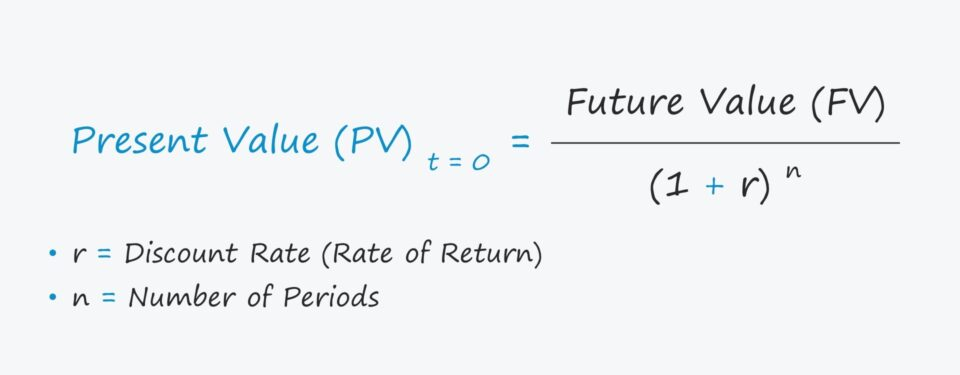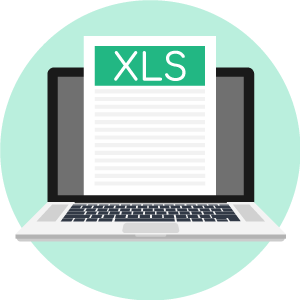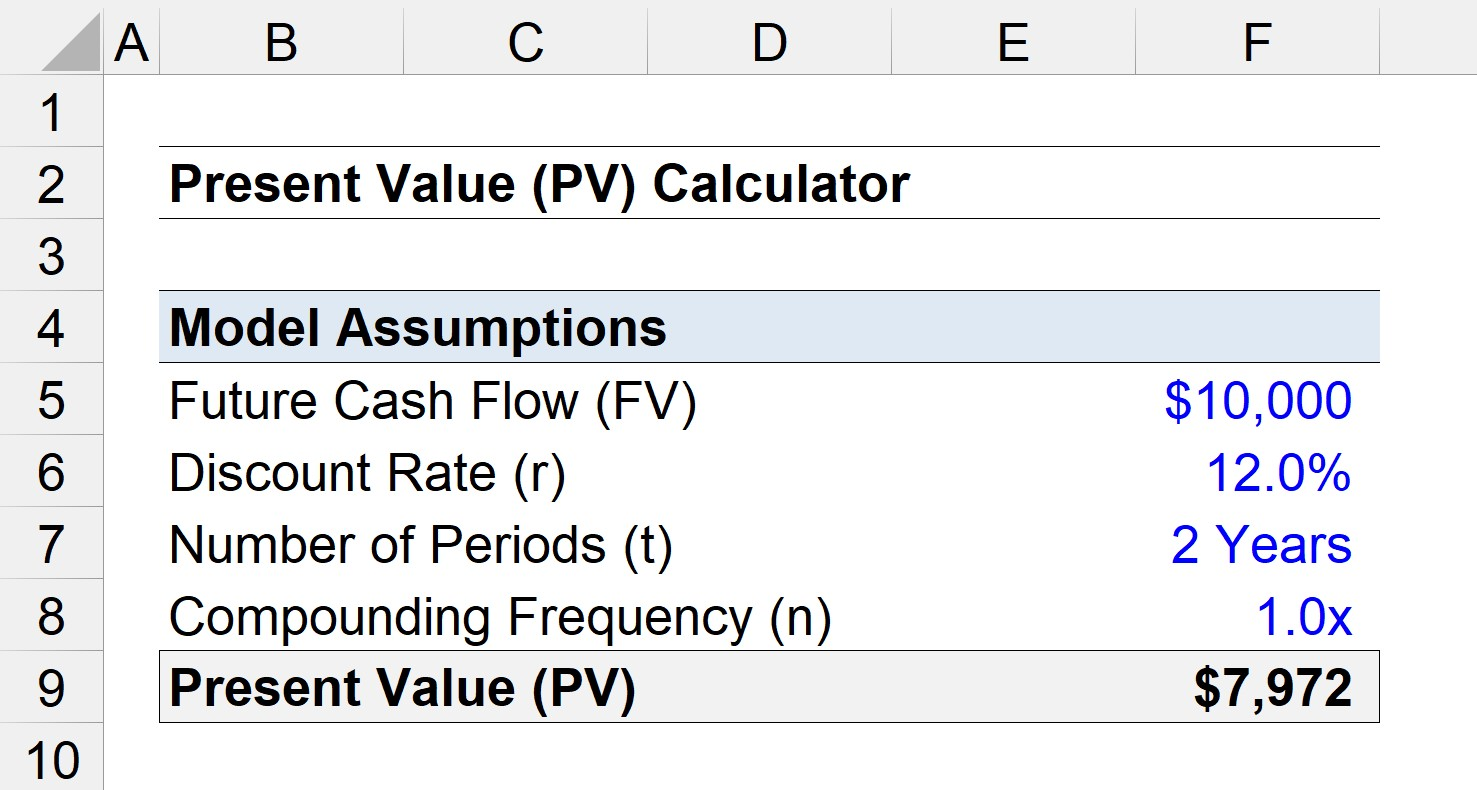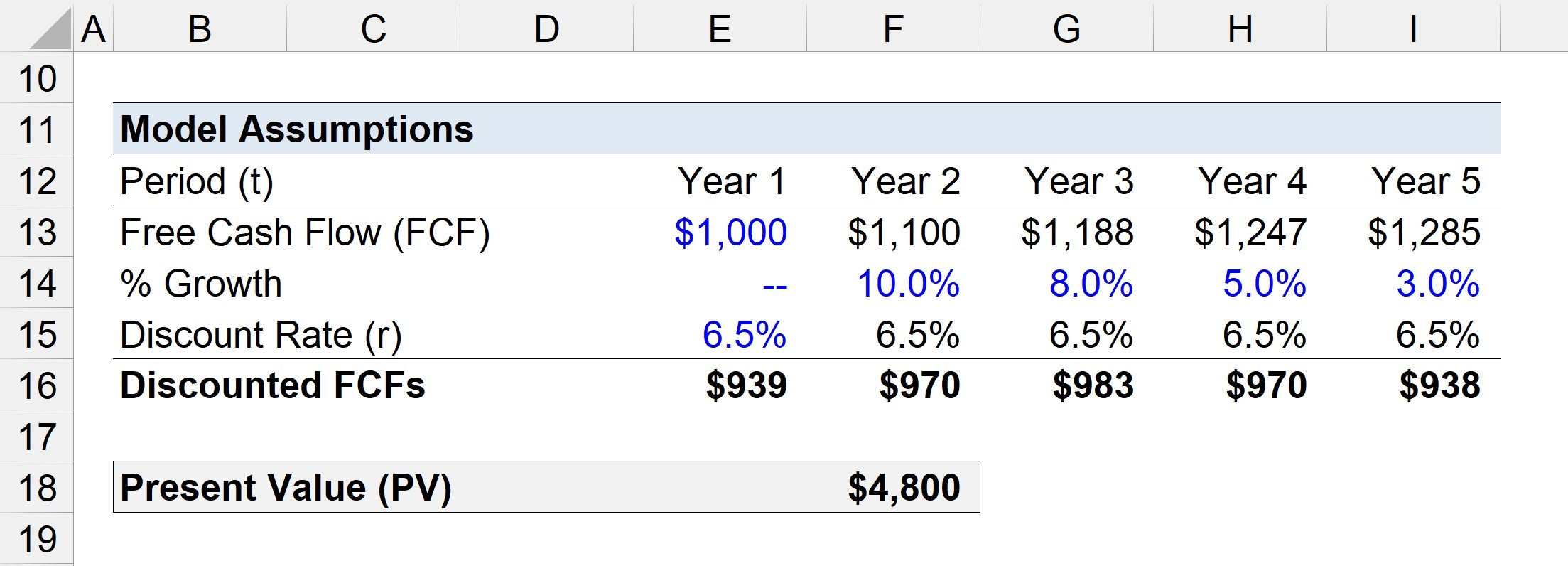# Present Value (PV)

Guide to Understanding the Present Value (PV) Concept• What is the present value (PV) formula?
• How is the PV concept tied to the intrinsic value (DCF) of a company?
• Which factors determine the present value (PV) of a cash flow?
• How is the present value different from the future value?

## Present Value (PV) Definition

The present value (PV) concept is fundamental to corporate finance and valuation.

The premise of the present value theory is based on the “time value of money”, which states that a dollar today is worth more than a dollar received in the future.

Therefore, receiving cash today is preferable (and more valuable) than receiving the same amount at some point in the future.

There are two primary reasons that support this theory:

1. Opportunity Cost of Capital: If the cash is currently in your possession, those funds could be invested into other projects to earn a higher return over time.
2. Inflation: Another risk to consider is the effects of inflation, which can erode the actual return on an investment (and thereby future cash flows lose value due to uncertainty).

Since money received on the present date carries more value than the equivalent amount in the future, future cash flows must be discounted to the current date when thought about in “present terms.”

Moreover, the size of the discount applied is contingent on the opportunity cost of capital (i.e. comparison to other investments with similar risk/return profiles).

All future receipts of cash (and payments) are adjusted by a discount rate, with the post-reduction amount representing the present value (PV).

Given a higher discount rate, the implied present value will be lower (and vice versa).

• Lower Discount Rate → Higher Valuation
• Higher Discount Rate → Lower Valuation
###### Intrinsic Value (DCF)

When estimating the intrinsic value of an asset, namely via the discounted cash flow (DCF) method, how much a company is worth is equal to the sum of the present value of all of the future free cash flows (FCFs) the company is expected to generate in the future.

More specifically, the intrinsic value of a company is a function of its ability to generate future cash flows and the risk profile of the cash flows, i.e. the company’s value is equal to the sum of the discounted values of its future free cash flows (FCFs).

## Present Value (PV) Formula

The present value (PV) formula discounts the future value (FV) of a cash flow received in the future to the estimated amount it would be worth today given its specific risk profile.

The formula used to calculate the present value (PV) divides the future value of a future cash flow by one plus the discount rate raised to the number of periods, as shown below.

###### PV Formula
• Present Value (PV) = FV / (1 + r) ^ n

Where:

• FV = Future Value
• r = Rate of Return
• n = Number of Periods
• Future Value (FV): The future value (FV) is the projected cash flow expected to be received in the future, i.e. the cash flow amount we are discounting to the present date.
• Discount Rate (r): The “r” is the discount rate – the expected rate of return (interest) – which is a function of the riskiness of the cash flow (i.e. greater risk → higher discount rate).
• Number of Periods (n): The final input is the number of periods (“n”), which is the duration between the date the cash flow occurs and the present date – and is equal to the number of years multiplied by the compounding frequency.

## Example of Present Value (PV) Calculation

Let’s say you loaned a friend \$10,000 and are attempting to determine how much to charge in interest.

If your friend has promised to repay the entire borrowed amount in five years, how much is the \$10,000 worth on the date the borrowed funds are returned?

Assuming that the discount rate is 5.0% – the expected rate of return on comparable investments – the \$10,000 in five years would be worth \$7,835 today.

• PV = \$10,000 /(1 + 5%)^5 = \$7,835

## Present Value vs. Future Value

The present value (PV) calculates how much a future cash flow is worth today, whereas the future value is how much a current cash flow will be worth on a future date based on a growth rate assumption.

While the present value is used to determine how much interest (i.e. the rate of return) is needed to earn a sufficient return in the future, the future value is usually used to project the value of an investment in the future.

• Present Value (PV) → How much is the future cash flow worth today?
• Future Value (PV) → How will this current cash flow be worth in the future?

## Present Value (PV) Calculator – Excel Template

We’ll now move to a modeling exercise, which you can access by filling out the form below.Submitting ...

## Present Value (PV) Example Calculation

Suppose we are calculating the present value (PV) of a future cash flow (FV) of \$10,000.

We’ll assume a discount rate of 12.0%, a time frame of 2 years, and a compounding frequency of one.

• Future Cash Flow (FV) = \$10,000
• Discount Rate (r) = 12.0%
• Number of Period (t) = 2 Years
• Compounding Frequency (n) = 1x

Using those assumptions, we arrive at a PV of \$7,972 for the \$10,000 future cash flow in two years.

• PV = \$10,000 / (1 + 12%)^(2*1) = \$7,972## Simple Discounted Cash Flow Exercise

In the next part, we’ll discount five years of free cash flows (FCFs).

Starting off, the cash flow in Year 1 is \$1,000 and the growth rate assumptions are shown below, along with the forecasted amounts.

• Year 1 = \$1,000
• Year 2 = 10% YoY Growth → \$1,100
• Year 3 = 8% YoY Growth → \$1,188
• Year 4 = 5% YoY Growth → \$1,247
• Year 5 = 3% YoY Growth → \$1,285

If we assume a discount rate of 6.5%, the discounted FCFs can be calculated using the “PV” Excel function.

• Year 1 = \$939
• Year 2 = \$970
• Year 3 = \$983
• Year 4 = \$970
• Year 5 = \$938

The sum of all the discounted FCFs amounts to \$4,800, which is how much this five-year stream of cash flows is worth today.Step-by-Step Online Course

#### Everything You Need To Master Financial Modeling

Enroll in The Premium Package: Learn Financial Statement Modeling, DCF, M&A, LBO and Comps. The same training program used at top investment banks.Inline FeedbacksLearn Financial Modeling Online

Everything you need to master financial and valuation modeling: 3-Statement Modeling, DCF, Comps, M&A and LBO.

X

The Wall Street Prep Quicklesson Series

7 Free Financial Modeling Lessons

Get instant access to video lessons taught by experienced investment bankers. Learn financial statement modeling, DCF, M&A, LBO, Comps and Excel shortcuts.